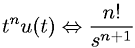Equations > Signal Processing > Laplace Transform Pairs > Laplace transform involving the unit step function

### Laplace transform involving the unit step functionLatex Code:

MathML Code:

 ${t}^{n}u\left(t\right)⇔\fracn!{s}^{n+1}$

MathType 5.0: